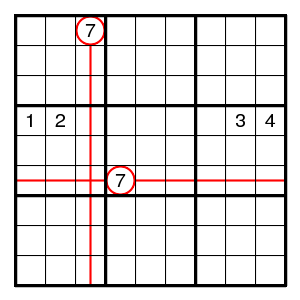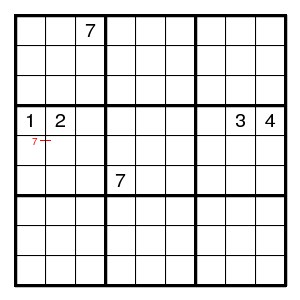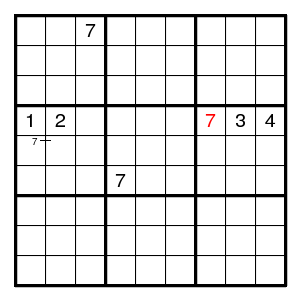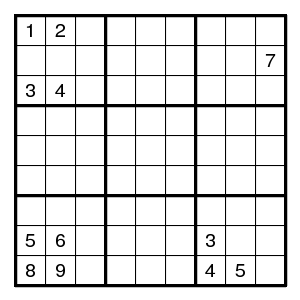Prev Up Next

# Forced pairs

A sudoku is called easy if it can be solved using only the techniques mentioned thus far. An easy sudoku can be solved step by step, where at each step there is only a unique possibility. It is not necessary to annotate the diagram. To find the next step in an easy sudoku, it suffices to check 324 things: 81 positions, 81 row-digit combinations, 81 column-digit combinations, and 81 box-digit combinations.

Terminology An easy sudoku is called 'solvable by singles only'.

In general one will want to annotate the diagram while solving it. That is more efficient (it saves repeated rechecking the same things) and allows more powerful arguments.

The most useful type of annotation is the pair. If examination of where a digit has to be in some row, column or box does not yield a unique position, but two possibilities, then write a small line and the digit involved. E.g., inone sees that there are two possibilities for the 7 in the middle left box, and writesNow it is clear that the 7 in the middle right box is uniquely determined:Terminology A pair is variously called 'strong pair', 'conjugate pair', 'bilocation'. Do not confuse a pair with a double pair (see below) or 2-set.

## Exercise

Which digit can be filled in the (partially given) grid below?